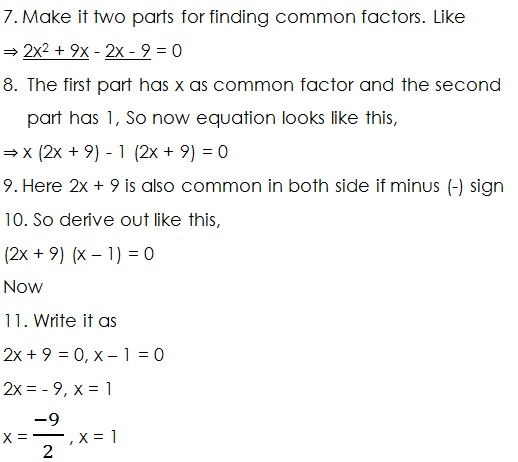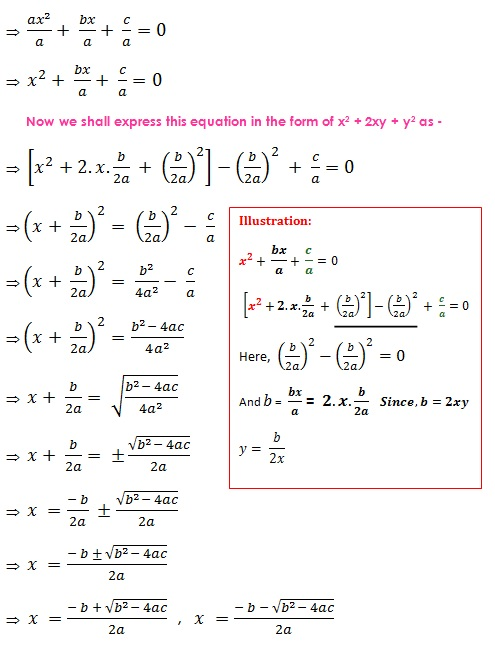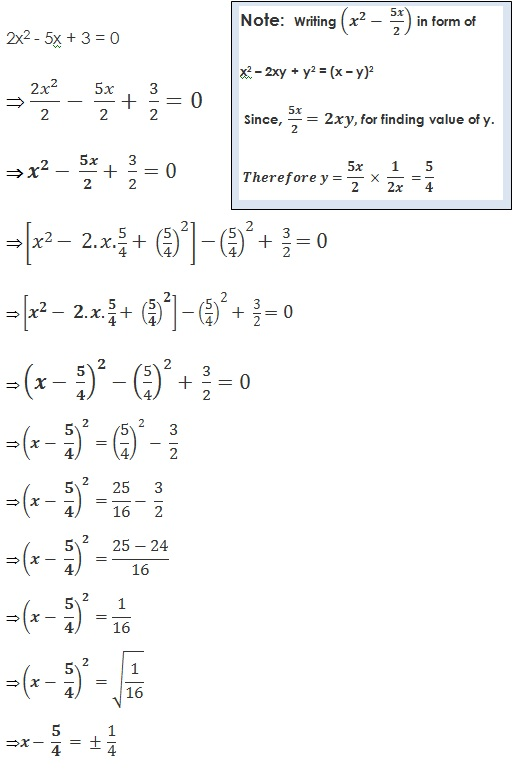Study Materials: CBSE Notes

Our CBSE Notes for Solution of Quadratic Equations Class 10 maths 4. Quadratic Equations - CBSE Notes - Toppers Study is the best material for English Medium students cbse board and other state boards students.

Notes ⇒ Class 10th ⇒ Mathematics ⇒ 4. Quadratic Equations

# Solution of Quadratic Equations Class 10 maths 4. Quadratic Equations - CBSE Notes - Toppers Study

Topper Study classes prepares CBSE Notes on practical base problems and comes out with the best result that helps the students and teachers as well as tutors and so many ecademic coaching classes that they need in practical life. Our CBSE Notes for Solution of Quadratic Equations Class 10 maths 4. Quadratic Equations - CBSE Notes - Toppers Study is the best material for English Medium students cbse board and other state boards students.

## Solution of Quadratic Equations Class 10 maths 4. Quadratic Equations - CBSE Notes - Toppers Study

CBSE board students who preparing for class 10 ncert solutions maths and Mathematics solved exercise chapter 4. Quadratic Equations available and this helps in upcoming exams 2023-2024.

### You can Find Mathematics solution Class 10 Chapter 4. Quadratic Equations

• All Chapter review quick revision notes for chapter 4. Quadratic Equations Class 10
• NCERT Solutions And Textual questions Answers Class 10 Mathematics
• Extra NCERT Book questions Answers Class 10 Mathematics
• Importatnt key points with additional Assignment and questions bank solved.

NCERT Solutions do not only help you to cover your syllabus but also will give to textual support in exams 2023-2024 to complete exercise maths class 10 chapter 4 in english medium. So revise and practice these all cbse study materials like class 10 maths chapter in english ncert book. Also ensure to repractice all syllabus within time or before board exams for ncert class 10 maths ex in english.

See all solutions for class 10 maths chapter 4 exercise 4 in english medium solved questions with answers.

### Solution of Quadratic Equations class 10 Mathematics Chapter 4. Quadratic Equations

• Solution Of Quadratic Equations Class 10 Maths 4. Quadratic Equations - CBSE Notes - Toppers Study
• Class 10 Ncert Solutions
• Solution Chapter 4. Quadratic Equations Class 10
• Solutions Class 10

## Solution of Quadratic Equations Class 10 maths 4. Quadratic Equations - CBSE Notes - Toppers Study

There are three method to find solution of quadratic equation.

(A)  By Factorisation Method:

(B)  By Completing Square Method:

Factorisation Method: In this method the middle term is seperated into two part and then there is found the two common factors.(B) Completing Square Method:

(i) Using Division

(ii) Using Multiplication

(i) Using Division:

Example 1:

Solving quadratic equation ax2 + bx + c = 0 for x

Dividing the equation by a.(i) Using Division

Example 2: Find the roots of the equation 2x2 – 5x + 3 = 0 by the method of completing the square.

Solution:(ii) Using multiplication:

Example 2. Solve for x using complete squaring method 2x2 + 3x - 7 = 0;##### Other Pages of this Chapter: 4. Quadratic Equations

Important Study materials for classes 06, 07, 08,09,10, 11 and 12. Like CBSE Notes, Notes for Science, Notes for maths, Notes for Social Science, Notes for Accountancy, Notes for Economics, Notes for political Science, Noes for History, Notes For Bussiness Study, Physical Educations, Sample Papers, Test Papers, Mock Test Papers, Support Materials and Books.Mathematics Class - 11th

NCERT Maths book for CBSE Students.

books

## Study Materials List:

##### Notes ⇒ Class 10th ⇒ Mathematics
1. Real Numbers
2. Polynomials
3. Pair of Linear Equations in Two Variables
5. Arithmetic Progressions
6. Triangles
7. Coordinate Geometry
8. Introduction to Trigonometry
9. Some Applications of Trigonometry
10. Circles
11. Constructions
12. Areas Related to Circles
13. Surface Areas and Volumes
14. Statistics
15. Probability

New Books